Two-order Runge-Kutta Method for systems of ordinary differential equations using Mathematica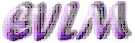Аnotation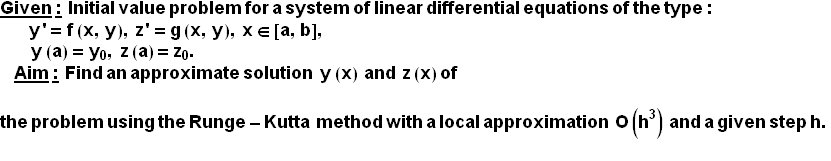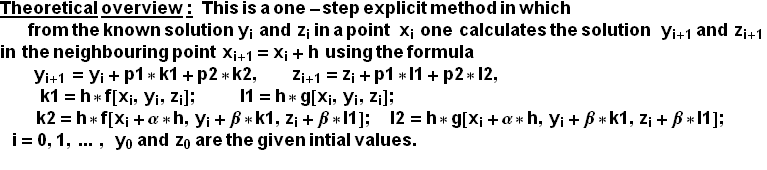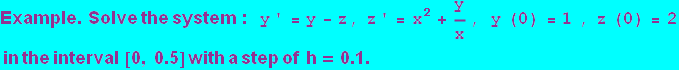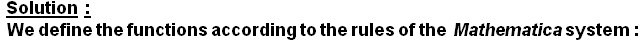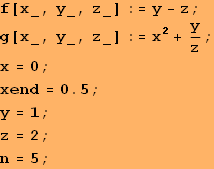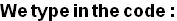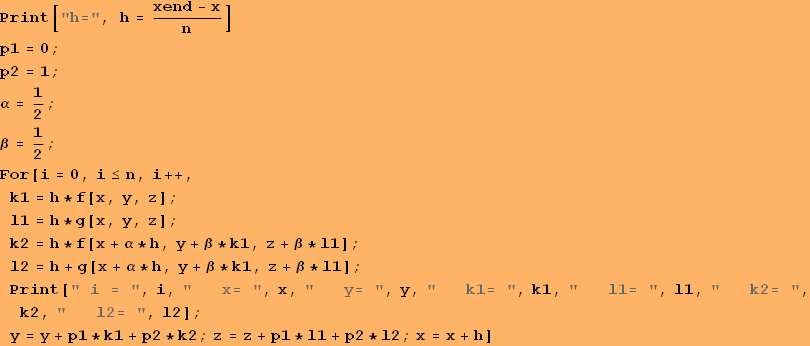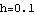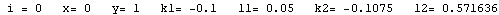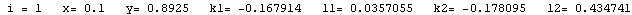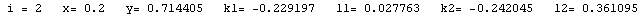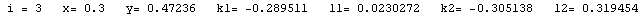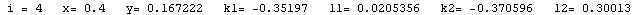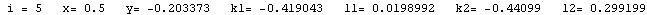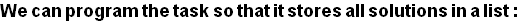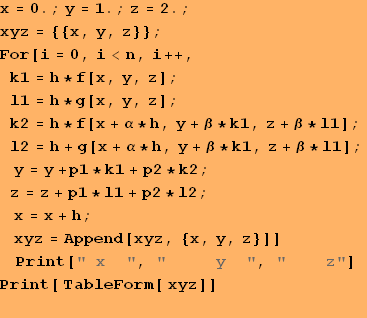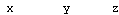0 1 2 0.1 0.8925 2.57164 0.2 0.714405 3.00638 0.3 0.47236 3.36747 0.4 0.167222 3.68692 0.5 -0.203373 3.98705

Conclusion:   The solution derived using the Runge-Kutta method O(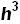) is in the form of a table of the variable functions y(x) and z(x), shown in the second and third columns of the table above, respectively.  Since the  local apriori error of the method is O() (or global error is  O(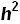) ) and here h=0.1, then these solutions must be rounded to three symbols after the decimal point.

Graphic of the solution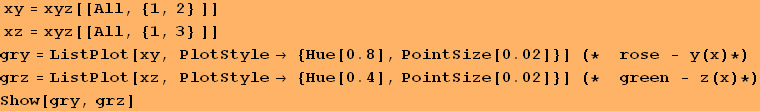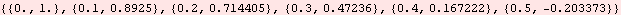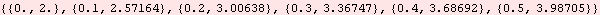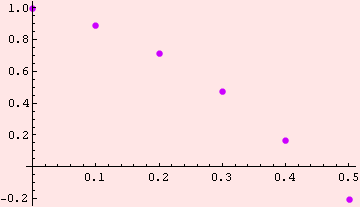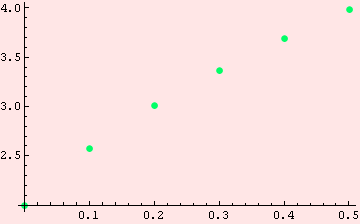Created by Wolfram Mathematica 6.0  (22 September 2008)